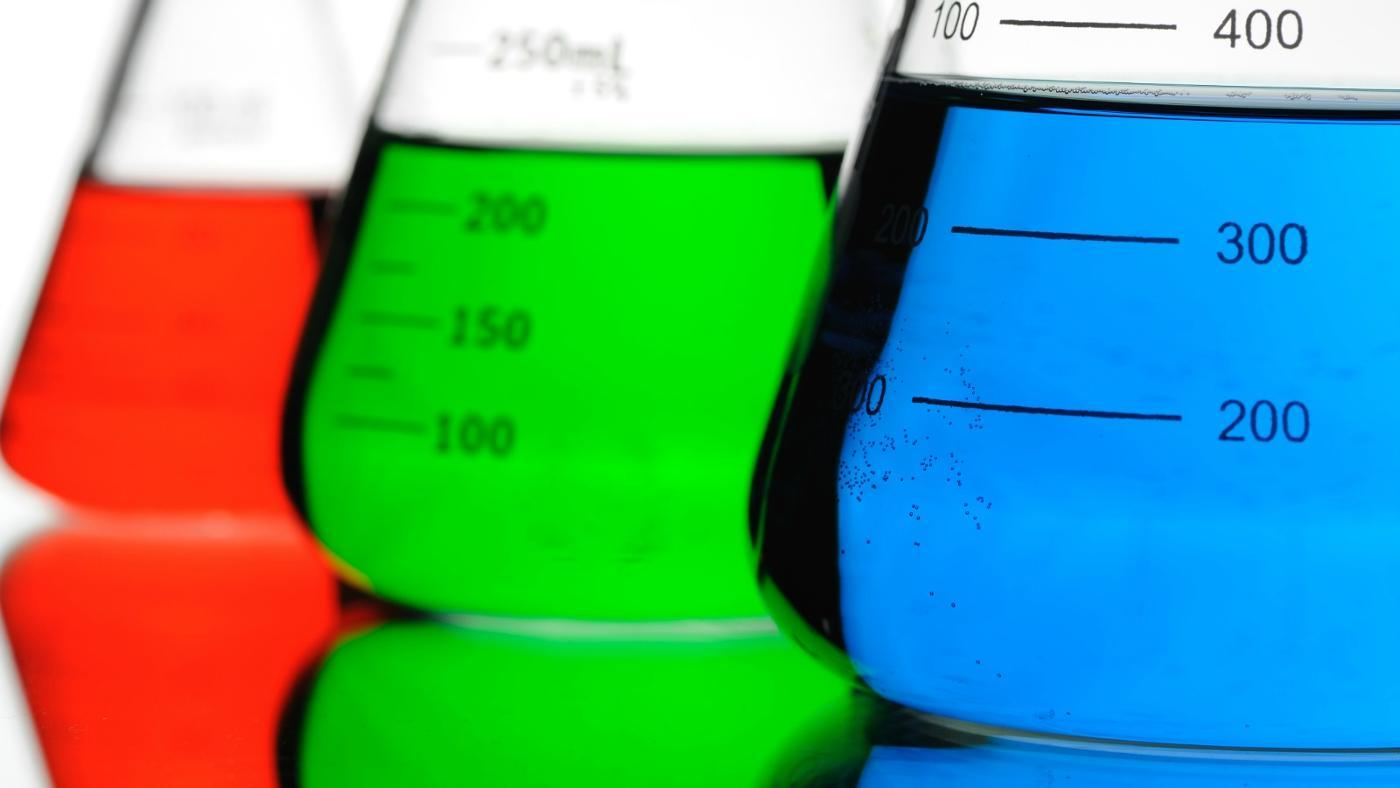Ads! Gain 200 Level Admission to Study Any Course In Any University Of Your Choice Through IJMB/JUPEB. NO JAMB | LOW FEES. Registration In Progress. Call 07063900993 NOW!

# Notable Differences Between Volume and Capacity You didn’t Notice

Filed in Education on December 3, 2020

Notable Differences Between Volume and Capacity You didn’t Notice.

Difference Between Volume and Capacity: Within the field of science, volume and capacity are easily mistaken and used interchangeably but they are different. Volume refers to the amount of three-dimensional space that it occupies while capacity is the maximum limits measured in liters.

Whenever volume and capacity are used, it usually involves an object and a specific amount of substance it contains which is the primary reason why the terms are assumed to be the same.## What is Volume?

Regardless of the state of matter involved (solid, liquid or gas), and whether or not there is actually an object within the container, the volume only refers to the three-dimensional space an object occupies by itself.

In other words, volume signifies the three-dimensional size of the object. It is determined as the product of the cross-sectional area and height of an object. Volume is commonly measured in terms of cubic meters or cubic centimeters.

At times, the volume of a certain container is also viewed as its capacity, as well. In the following figure, the volume of the cylinder is equal to the product of cross-sectional area A and height h; i.e V=A×h.

Fluid displacement is another way to determine the volume of solids or gas. Fluid displacement involves immersing the object in a fluid. The volume of the object will displace the fluid. Such displacement of the fluid can be measured. In that case, it will be expressed in milliliters, liters, fluid ounces, or cups.

The volume of fluids, or a quantity of small loose objects such as grains of rice, can be measured by pouring them into a measuring tool such as a measuring cup

## What is Capacity?

It refers to the potential amount of substance that a container is able to hold or absorb. It may have similarities in ideas with volume, but it is still distinguishable.

Capacity concentrates more on how much a solid, liquid or gas can fit in a container and often implies the maximum amount that one can bear. Capacity is usually measured in terms of liters, milliliters, pound, gallons, and the like. For example, in the figure shown below, the capacity of the measuring cup is 250 ml.

One way to illustrate the concept is that capacity is the ability of a container to hold two cups of rice, while that same container may have a volume of 5 cubic centimeters which refers to the amount of space that the container itself occupies.

## Distinction Between Volume and Capacity

1. Volume is the amount of space taken up by an object, while capacity is the measure of an object’s ability to hold a substance, like a solid, a liquid or a gas.

2. Capacity is the maximum limit measured in liters.

3. The Volume is measured in cubic units, while capacity can be measured in almost every other unit.

4. Volume is calculated by multiplying the length, width, and height of an object, while capacity’s measurement is geared more towards cc or ml.

CSN Team.

Delivered by TMLT NIGERIA